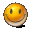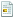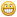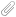# WC3 Modding Information CenterFor your WC3 needs...

 Warcraft III: Maps Models Skins Icons Spells / Systems Tools TutorialsChat @Discord

### JAVAGame Development

0 Members and 1 Guest are viewing this topic.

##### JAVA
on: January 13, 2013, 11:30:06 AM

Code: Text
1. package org.moyack;
2.
3. public class Main {
4.
5.         public static void main(String[] args) {
6.                 int a = 10;
7.                 int b = 3;
8.                 System.out.println(max(a, b));
9.         }
10.
11.         static int max(int a, int b) {
12.                 if (a > b) {
13.                         return a;
14.                 } else {
15.                         return b;
16.                 }
17.         }
18.
19. }
20.

##### JAVA
Reply #1 on: January 13, 2013, 11:52:04 AM

Code: Text
1. package org.moyack;
2.
3. import java.io.IOException;
4. import java.util.Scanner;
5.
6. /**
7.  * An example program for reading data from standard input
8.  * */
9. public class Main {
10.
11.         public static void main(String[] args) throws IOException {
12.                 // create scanner of system input
13.                 Scanner scanner = new Scanner(System.in);
14.
15.                 // define integer and real variables
16.                 int i = 0;
17.                 double d = 0.0;
18.
19.                 // print the prompt for user
20.                 System.out.print("i=");
21.                 // read integer variable from console
22.                 // (click down in console window and type in the number)
23.                 i = scanner.nextInt();
24.
25.                 // print the prompt for user
26.                 System.out.print("d=");
28.                 d = scanner.nextDouble();
29.
30.                 // print out to see if everything is ok
31.                 System.out.println("i=" + i + ", d=" + d);
32.
33.                 // close the scanner to prevent memory leak
34.                 scanner.close();
35.         }
36. }
37.

##### JAVA
Reply #2 on: January 13, 2013, 12:04:36 PM

Ok here is some homework.

1. Find the sum of odd numbers from a to b (you enter a and b on the console)

2. Enter the radius and height of a cylinder and print out the volume. (http://www.mathopenref.com/cylindervolume.html)
TIP: use java constant Math.PI

##### JAVA
Reply #3 on: January 14, 2013, 10:51:14 AM

I've installed eclipse in my linux netbook and I plan to do the homework in my free time @school. One question is:

can I do the homework with vanilla Eclipse??

##### JAVA
Reply #4 on: January 14, 2013, 11:36:28 AM

Yes, we are doing plain java now.

Mobile part is just a plugin for eclipse, we will do that once you get familiar enough with basics of language.

Here are some tutorials
http://www.tutorialspoint.com/java/index.htm
« Last Edit: January 15, 2013, 02:19:30 PM by cohadar »

##### JAVA
Reply #5 on: January 17, 2013, 10:31:50 PM

The homework thus far:

Code: Text
1. package moyack.org;
2.
3. import java.io.IOException;
4. import java.util.Scanner;
5.
6. public class moyack {
7.
8.         /**
9.          * @param args
10.          */
11.         public static void main(String[] args) throws IOException {
12.                 // create scanner of system input
13.                 Scanner scanner = new Scanner(System.in);
14.
15.                 // define integer and real variables
16.                 int i = 0;
17.                 int j = 0;
18.                 double t = 0.0;
19.                 double t2 = 0.0;
20.                 while (i >= j) {
21.                         System.out.println("Please enter the values in order (from minor to mayor)...");
22.                         System.out.print("first value=");
23.                         i = scanner.nextInt();
24.                         System.out.print("second value=");
25.                         j = scanner.nextInt();
26.                         if (i > j) {
27.                         }
28.                 }
29.                 t = i/2;
30.                 t2 = Math.round(i/2);
31.                 System.out.println("t=" + t + " ; t2=" + t2);
32.
33.                 // close the scanner to prevent memory leak
34.                 scanner.close();
35.
36.         }
37.
38. }
39.

##### JAVA
Reply #6 on: January 19, 2013, 04:57:08 PM

Not like that.
You enter a and b (just 2 numbers) and sum the odd numbers between them (by using some loop)

For example if you enter 4 and 13 your sum is = 5+7+9+11+13
tip: use if inside a loop to separate even and odd numbers

##### JAVA
Reply #7 on: January 20, 2013, 10:17:43 PM

Not like that.
You enter a and b (just 2 numbers) and sum the odd numbers between them (by using some loop)

For example if you enter 4 and 13 your sum is = 5+7+9+11+13
tip: use if inside a loop to separate even and odd numbers

Working on it, sorry for not talking today, I had to do a lot of stuff preparing the classes initiation. Hard working now to ensure free time from the rest of the year... well, sort of...

EDIT: first homework done...
Code: Text
1. package org.moyack;
2.
3. import java.io.IOException;
4. import java.util.Scanner;
5.
6. public class Main {
7.
8.         /**
9.          * @param args
10.          */
11.         public static void main(String[] args) throws IOException {
12.                 // create scanner of system input
13.                 Scanner scanner = new Scanner(System.in);
14.
15.                 // define integer and real variables
16.                 int i = 0;
17.                 int j = 0;
18.                 int k = 0;
19.                 double t = 0.0;
20.                 double t2 = 0.0;
21.                 while (i >= j) {
22.                         System.out
23.                                         .println("Please enter the values in order (from minor to mayor)...");
24.                         System.out.print("first value=");
25.                         i = scanner.nextInt();
26.                         System.out.print("second value=");
27.                         j = scanner.nextInt();
28.                 }
29.                 t = i / 2.0;
30.                 t2 = Math.round(i / 2.0);
31.                 if (t == t2) {
32.                         i = i + 1;
33.                 }
34.                 t = j / 2.0;
35.                 t2 = Math.round(j / 2.0);
36.                 if (t == t2) {
37.                         j = j - 1;
38.                 }
39.                 k = i;
40.                 while (i + 2 <= j) {
41.                         i = i + 2;
42.                         k = k + i;
43.                 }
44.                 System.out
45.                                 .println("The sum of all odd numbers from the numbers indicated is: "
46.                                                 + k);
47.                 // close the scanner to prevent memory leak
48.                 scanner.close();
49.
50.         }
51. }
52.

Second EDIT: The other homework doneCode: Text
1. package org.moyack;
2.
3. import java.io.IOException;
4. import java.util.Scanner;
5.
6. public class Main {
7.
8.         /**
9.          * @param args
10.          */
11.         public static void main(String[] args) throws IOException {
12.                 // create scanner of system input
13.                 Scanner scanner = new Scanner(System.in);
14.
15.                 // define integer and real variables
16.                 double r = 0.0;
17.                 double h = 0.0;
18.                 while (r <= 0 || h <= 0) {
19.                         System.out
20.                                         .println("Please enter the values of radius and height of the cylinder...");
21.                         System.out.println("(These values MUST BE POSITIVE!!!)");
23.                         r = scanner.nextDouble();
24.                         System.out.print("height=");
25.                         h = scanner.nextDouble();
26.                 }
27.                 System.out.println("the volume of the cylinder is: " + Math.PI * r * r
28.                                 * h);
29.                 scanner.close();
30.
31.         }
32. }
33.
« Last Edit: January 20, 2013, 11:24:03 PM by moyack »

##### JAVA
Reply #8 on: January 21, 2013, 02:50:07 AM

Ok, these programms are "correct" but they are not "good".
Why?

Because they have more stuff than they need to.

Useless local variables
Code: Text
1.                 int k = 0;
2.                 double t = 0.0;
3.                 double t2 = 0.0;
4.

Useless code (delete your experiment code)
Code: Text
1.                 t = i / 2.0;
2.                 t2 = Math.round(i / 2.0);
3.                 if (t == t2) {
4.                         i = i + 1;
5.                 }
6.                 t = j / 2.0;
7.                 t2 = Math.round(j / 2.0);
8.                 if (t == t2) {
9.                         j = j - 1;
10.                 }
11.

Also there is no need to put WHILE around inputs - if user fucks input let the brogram break, not your problem atm.

You need to do both homeworks again.
But this time do it from zero, no copy paste of whole blocks of code.
Make new class for each homework! Homework1, Homework2
And make those classes in org.moyack.homework package
And when you make the homework make sure you write proper comments to explain what code does,
and make sure to delete any bad comments that are left over from copy paste.

Any fool can write code that a computer can understand.  Good programmers write code that humans can understand.
~Martin Fowler

##### JAVA
Reply #9 on: January 27, 2013, 10:01:47 AM

Ok... I've done the fixes...

Summing odd numbers...
Code: Text
1. package org.moyack;
2.
3. // Code to sum the odd numbers between 2 integers...
4.
5. import java.io.IOException;
6. import java.util.Scanner;
7.
8. public class homework1 {
9.
10.         /**
11.          * @param args
12.          */
13.         public static void main(String[] args) throws IOException {
14.                 Scanner scanner = new Scanner(System.in);
15.                 int i = 0;
16.                 int j = 0;
17.                 int k = 0;
18.                 System.out
19.                                 .println("Please enter the values in order (from minor to mayor)...");
20.                 System.out.print("first value="); // first argument
21.                 i = scanner.nextInt();
22.                 System.out.print("second value="); // second argument
23.                 j = scanner.nextInt();
24.                 // finish the code if the data is entered wrong...
25.                 if (i >= j) {
26.                         System.err
27.                                         .println("first value is bigger or equal than the second value... exit...");
28.                         scanner.close();
29.                         return;
30.                 }
31.                 // checks if i and j are even numbers... if so, they're set to the nearest odd number
32.                 if (i / 2.0 == Math.round(i / 2.0)) {
33.                         i = i + 1;
34.                 }
35.                 if (j / 2.0 == Math.round(j / 2.0)) {
36.                         j = j - 1;
37.                 }
38.                 // sums the values between i and j...
39.                 k = i;
40.                 while (i + 2 <= j) {
41.                         i = i + 2;
42.                         k = k + i;
43.                 }
44.                 // prints out the result...
45.                 System.out
46.                                 .println("The sum of all odd numbers from the numbers indicated is: "
47.                                                 + k);
48.                 scanner.close();
49.         }
50.
51. }
52.

Calculating cylinder...
Code: Text
1. package org.moyack;
2.
3. import java.io.IOException;
4. import java.util.Scanner;
5.
6. public class homework2 {
7.
8.         /**
9.          * @param args
10.          */
11.         public static void main(String[] args) throws IOException {
12.                 // those are the values for the cylinder
13.                 double r = 0.; // radius...
14.                 double h = 0.; // height...
15.                 Scanner scanner = new Scanner(System.in);
16.                 System.out
17.                                 .println("Please enter the values of radius and height of the cylinder...");
18.                 System.out.println("(These values MUST BE POSITIVE!!!)");
20.                 r = scanner.nextDouble();
21.                 System.out.print("height=");
22.                 h = scanner.nextDouble();
23.                 if (r <= 0 || h <= 0) { // checks if r or h are negative values or equal
24.                                                                 // to 0...
25.                         System.err
26.                                         .println("radius or height are less or equal to 0... exit...");
27.                         scanner.close();
28.                         return;
29.                 }
30.                 // the formulae is calculated directly in the println() function...
31.                 // testing Math.pow... :)
32.                 System.out.println("the volume of the cylinder is: " + Math.PI
33.                                 * Math.pow(r, 2.0) * h);
34.                 scanner.close();
35.         }
36.
37. }
38.
« Last Edit: January 27, 2013, 10:20:38 AM by moyack »

##### JAVA
Reply #10 on: January 28, 2013, 02:55:11 AM

Very good Moyack.

Just one detail.

Code: Text
1. // this is not an efficient way to check if number is even
2. if (i / 2.0 == Math.round(i / 2.0)) {
3.

Code: Text
1. // this is how you check if number is even
2. if (i % 2 == 0) {
3.

Code: Text
1. // this is how you check if number is odd
2. if (i % 2 != 0) {
3.

Code: Text
1. // always compare with zero, never with 1 because % can return -1 for negative i
2. if (i % 2 == 1) {   // VERY BAD
3.

Ok now try some online tutorials. When you get to the point where you need more help ask here and I will clarify.

http://www.tutorialspoint.com/java/index.htmStarted by Purgeandfire Replies: 0Views: 1734 Tutorial ZoneStarted by moyack Replies: 1Views: 1844 Site DiscussionStarted by olofmoleman Replies: 0Views: 693 Warcraft III ModelsStarted by REDSEW Replies: 2Views: 8615 Site DiscussionStarted by olofmoleman Replies: 0Views: 937 Warcraft III Models